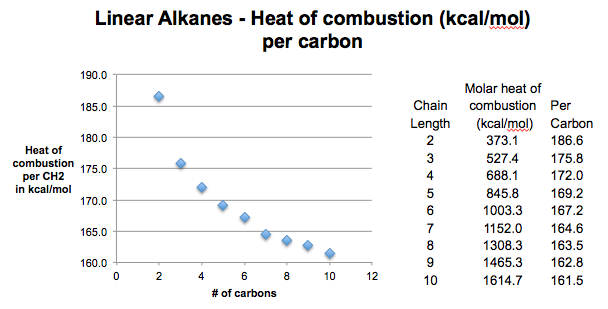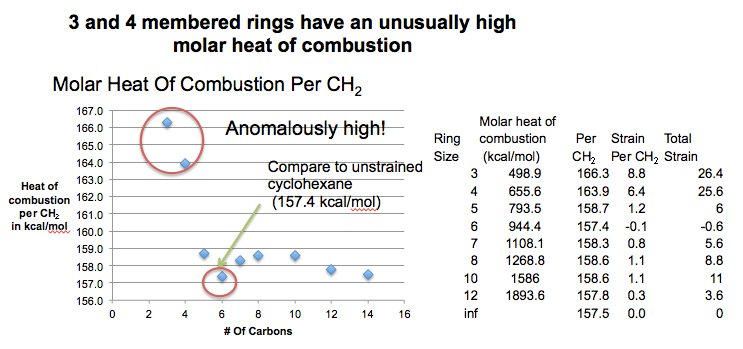# Calculation of Ring Strain In Cycloalkanes

Last updated: August 4th, 2020 |

Ring Strain In Cycloalkanes (1) – Calculation of Ring Strain

This post is all about how ring strain is calculated. If you want more specific details about ring strain in cyclopropane and cyclobutane, I suggest moving on to the next post.

In the last post we learned about one consequence of the fact that carbon can form rings – that we can form stereoisomers (cis / trans).  This post attempts to explain another very interesting consequence of ring formation: ring strain.

It all starts with this: it turns out you can learn a lot about molecules by burning them. In a controlled and quantified way, of course. : – )

## 1. Heat of Combustion Of Alkanes

The molar heat of combustion, as you might recall from gen chem, is the energy released upon burning one mole of a substance. It’s a value of enthalpy [Δ H] – usually measured in kJ/mol or kcal/mol. We’re going to use kcal/mol here [why do organic chemists use kilocalories] but to convert to kJ/mol, multiply by 4.184 and you get the same thing.

Let’s start with something simple. Imagine we had a series of straight chain alkanes. Ethane, propane, butane, all the way up to dodecane (12).

As we increase the number of carbons, the increase energy released upon combustion will increase. I like to use the analogy of an infinite staircase: with every step we go higher, we’re increasing the distance between us and the floor, and therefore increasing the energy released if we were to fall from the staircase down to the ground : – ) .## 2. Heat of Combustion As A Function Of Chain Length

Let’s ask a simple question: how does the heat of combustion change as we add extra carbons?
Do you think we would we expect to see an “equal spacing” of energies as we successively add a carbon (by analogy to a staircase with equally spaced steps)? Or would the spacing gradually change?  And, importantly, could we use this information to make predictions?

Let’s burn various straight chain hydrocarbons and measure how much energy (in kcal/mol) is released by each molecule.
We can then calculate the average energy released per carbon – this gives us the “spacing” between each step. This will help us predict the energy released for the next step.

[Here, use your imagination as we burn hydrocarbons of varying length and measure the heat of combustion for each case].

Let’s now look at the data. When we do this, we see the kcal/mol per carbon starts high: 213 for methane (not shown) ,  186 (for ethane) and then starts reaching a limit a little bit lower than 160 kcal/mol. This is like a staircase where the steps start off large, but gradually decrease in height until they all become a uniform height.## 3. The Heat Of Combustion For A Very Long Straight Chain Alkane is About 157 kcal/mol Per CH2

What’s up with the curve, by the way? Very subtle question. Answer is long, so it’s in this note. Short version: in a straight chain alkane of formula  CH3(CH2)nCH3 , the heat released from burning the ends  [CH3] is higher than that obtained from burning the interior CH2 groups. As the chain gets longer, and the #CH2 >> #CH3 the relative contribution of the 2 CH3 groups to the overall ΔH will decrease. Therefore in the limit of infinite n the value of ΔHcombustion will approach that of CH2 (about 157 kcal/mol).

## 4. Cycloalkanes Have The General Formula (CH2)n

So what does this have to do with cycloalkanes? Cycloalkanes are composed only of CH2 groups. There’s no “ends” to worry about. So if  we repeated the same combustion experiment – starting with cyclopropane, and increasing CH2 by one each time, we might expect only to see heats of combustion that go up only by the ΔH of CH2 (157 kcal/mol).

In other words, we’d naively expect to see a staircase with uniform step height, all the way up.

Science often begins by having these naive notions about how nature should work, and then doing the experiment and finding that reality is contrary to (and thus much more interesting than) our expectations. This is a prime example!

## 5. Any Deviation From A Value Of 157 kcal/mol Per CH2 Tells Us About Ring Strain

Here’s what happens when we burn ‘em. Look at that “average step height” (aka ΔHcombustion/CH2).  It’s MUCH larger than we expect for C=3 (cyclopropane, 166.3 kcal/mol) and C=4 (cyclobutane, 163.9 kcal/mol), hits a minimum at C=6 (cyclohexane 157.4 kcal/mol) and then nudges up again until hitting another minimum at C=12 (cyclododecane). Not included in the graph, but the step height is constant from there on out, at about 157.4 kcal/mol.
So if we take 157.4 kcal/mol as a baseline, cyclopropane releases 8.8 “extra” kcal/mol per carbon, for a total of 26.4 kcal/mol. Cyclobutane releases 6.4 “extra” kcal/mol per carbon, for a total of 25.6 kcal/mol. This “extra” energy means these molecules are actually more unstable than we expected (“higher up the staircase” than we thought – so there’s farther to fall to the ground).  We call this “extra” instability, “strain”.Note – we’d get similar results if we compared heats of formation instead of heats of combustion, but combustion is so much more vivid : – )

## 6. What Factors Might Lead To Ring Strain?

Like any good experiment, this raises a whole lot of new questions.

• First, what could be a source of this “strain”? Why might cyclopropane and cyclobutane be much more unstable than we naively expected?
• Second, why is cyclohexane more unstrained than cyclopentane? For example, the interior angles of a pentagon (108°) are much closer to the ideal bond angles for a tetrahedron (109°) than a hexagon is (120°). So what gives?
• Third, what’s up with that increase in strain between C=6 (cyclohexane) and C=14 ? Why does strain go up from cyclohexane and then go back down again?

In the next post we’ll deal with cyclopropane and cyclobutane, and then future posts will go through the next two questions.

## Notes

NOTE: In excess O2, all C is converted to CO2 and all H is converted to H2O.  Burning  methane (CH4) gives us 1 equiv CO2 and 2 equivs of H2O.   Ethane gives us 2 equivs CO2 and 3 equivs of H2O. Propane gives us 3 equivs CO2 and 4 equivs H2O. On a per-carbon basis, as we increase the # of carbons, the CO2/H2O ratio will approach 1. The ΔHcombustion on a per-carbon basis is highest for methane because proportionately more H2O is formed, so therefore proportionately more energy is released. back to top

## Comment section

### 8 thoughts on “Calculation of Ring Strain In Cycloalkanes”

1.ritwika says:

Wonderful articles….vry easy to understand…!!!

2.Carlos says:

I tried to calculate the angle strain for cyclopropane and can not get 26.4.

1.Rutwik Dhale says:

It’s 24.75 and not 26.4

2.James Ashenhurst says:

3 times 8.8

3.Oosman Beekawoo says:

Why does cyclohexane have negative strain?

1.James says:

Cyclohexane has zero strain, not negative strain.

This site uses Akismet to reduce spam. Learn how your comment data is processed.